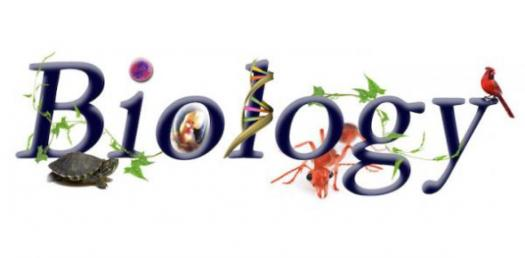# Principles Of Biology Chapter 2 And 3

44 Questions | Total Attempts: 1042SettingsThe study of biology may not be as flashy as chemistry or physics, but it’s one of the more interesting scientific topics to learn about if you’re somebody who has a passion for animals and the human race in general from a scientific standpoint. What are our bodies made up of, for example? Learn about all that and more in the following college-level quiz on the Principles of Biology.

• 1.
What charge does the subatomic particle proton have?
• A.

No charge

• B.

(+) positive

• C.

(-) negative

• 2.
What charge does the subatomic particle neutron have?
• A.

(-) negative

• B.

(+) positive

• C.

No charge

• 3.
What charge does the subatomic particle electron have?
• A.

No charge

• B.

(-) negative

• C.

(+) positive

• 4.
What are the subatomic particles?
• 5.
Which subatomic particle is involved with forming chemical bonds?
• A.

Neutron

• B.

Electron

• C.

Proton

• 6.
What are the levels of organization of living things?
• A.

Atom, Molecule, Class, Tissue, Organ, Organism, Population, Ecosystem, Bioshpere

• B.

Atom, Molecule, Cell, Tissue, Organ, Organ system, Organism, Population, Community, Evolution

• C.

Atom, Molecule, Cell, Tissue, Organ, Organ system, Organism, Population, Community, Ecosystem, Biosphere

• D.

Atom, Cell, Tissue, Organ system, Community, Biosphere

• 7.
What is the term for atoms that are electrically charged as a result of gaining or losing electrons?
• 8.
Which is the reactant?Ex.A+B ---> C
• A.

A

• B.

B

• C.

A+C

• D.

A+B

• E.

C

• 9.
Which is the product?Ex.A+B ---> C
• A.

A+B

• B.

C

• C.

A

• D.

B

• E.

B+C

• 10.
What is the process of smaller things built up to larger?Ex.A+B ---> C(Sm) (L)
• A.

Catabolic

• B.

Anabolic

• 11.
What is the process of larger things breaking down to smaller?Ex.C ---> A+B(L) (Sm)
• A.

Catabolic

• B.

Anabolic

• 12.
What elements make up water?
• A.

Oxygen

• B.

Hydrogen

• C.

H2O

• 13.
What type of bond holds the atoms of the molecule together?
• A.

Chemical bond

• B.

Hydrogen bond

• C.

Ionic bond

• D.

Covalent bond

• 14.
What are the properties of water?
• A.

Cohesion, surface tension

• B.

Temperature regulation, freezing properties

• C.

Solvent for biochemical reactions

• D.

All of the above

• E.

None of the above

• 15.
The fluids of organisms are _______ solutions.
• 16.
What is the tool used to measure the hydrogen ion (H+) concentration in a solution?
• A.

Acids

• B.

Bases

• C.

PH scale

• 17.
What is the chemical compound that releases H+ to a solution?
• A.

Bases

• B.

Acids

• C.

Buffers

• 18.
What compound accepts H+ and removes it from solution?
• A.

Acids

• B.

Bases

• C.

Buffers

• 19.
What pH level makes a solution chemically neutral?
• A.

Above 7

• B.

Below 7

• C.

7

• 20.
How many bonds does carbon form?
• A.

2

• B.

5

• C.

4

• D.

1

• E.

3

• 21.
How many bonds do hydrogen form?
• A.

3

• B.

1

• C.

4

• D.

2

• E.

5

• 22.
In correct order, what does HONC stand for?
• 23.
The organic molecules that make up a cell are very large. What are they called?
• 24.
What are the 4 macromolecules that make up the cell?
• A.

Carbon, hydrogen, oxygen, nitrogen

• B.

Proteins, carbohydrates, lipids, nucleic acids

• C.

None of the above

• 25.
What are the 4 elements that are most prevalent in biology?
• A.

Carbon dioxide, hydrogen, oxygen, nitrogen

• B.

Carbohydrates, lipids, proteins, nucleic acid

• C.

Carbon, proteins, oxygen, nucleic acid

• D.

Carbon, hydrogen, oxygen, nitrogen

Related TopicsBack to top